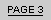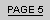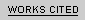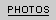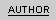M O D E R N I T Y   A N D   T H E   M A N I A CP a g e   F o u r :   T h e   S h o w e r   S c e n e M a r i o n   g e t s   a   v i s i t o r   w h i l e   s h o w e r i n g   a t   t h e   B a t e s   M o t e l.A R T I C L E   B Y   R I C H A R D   A R M S T R O N G